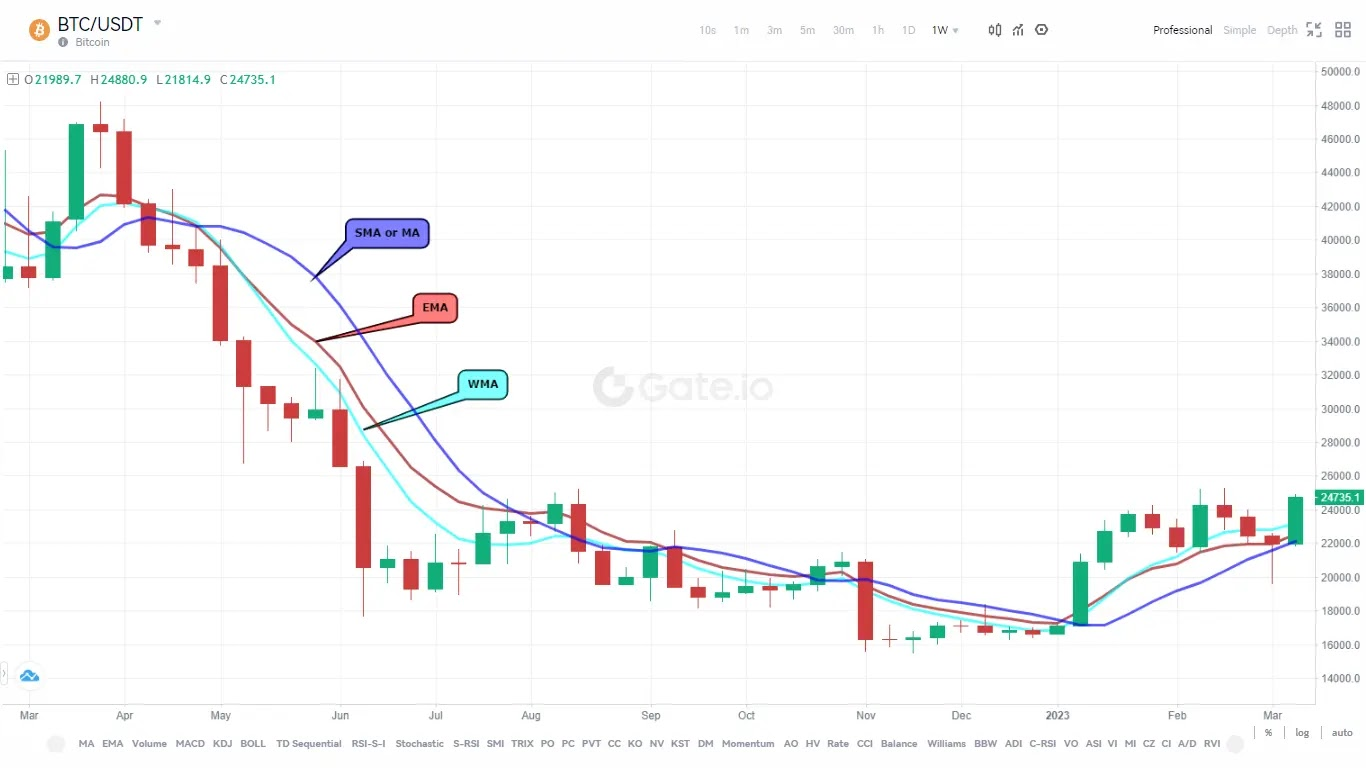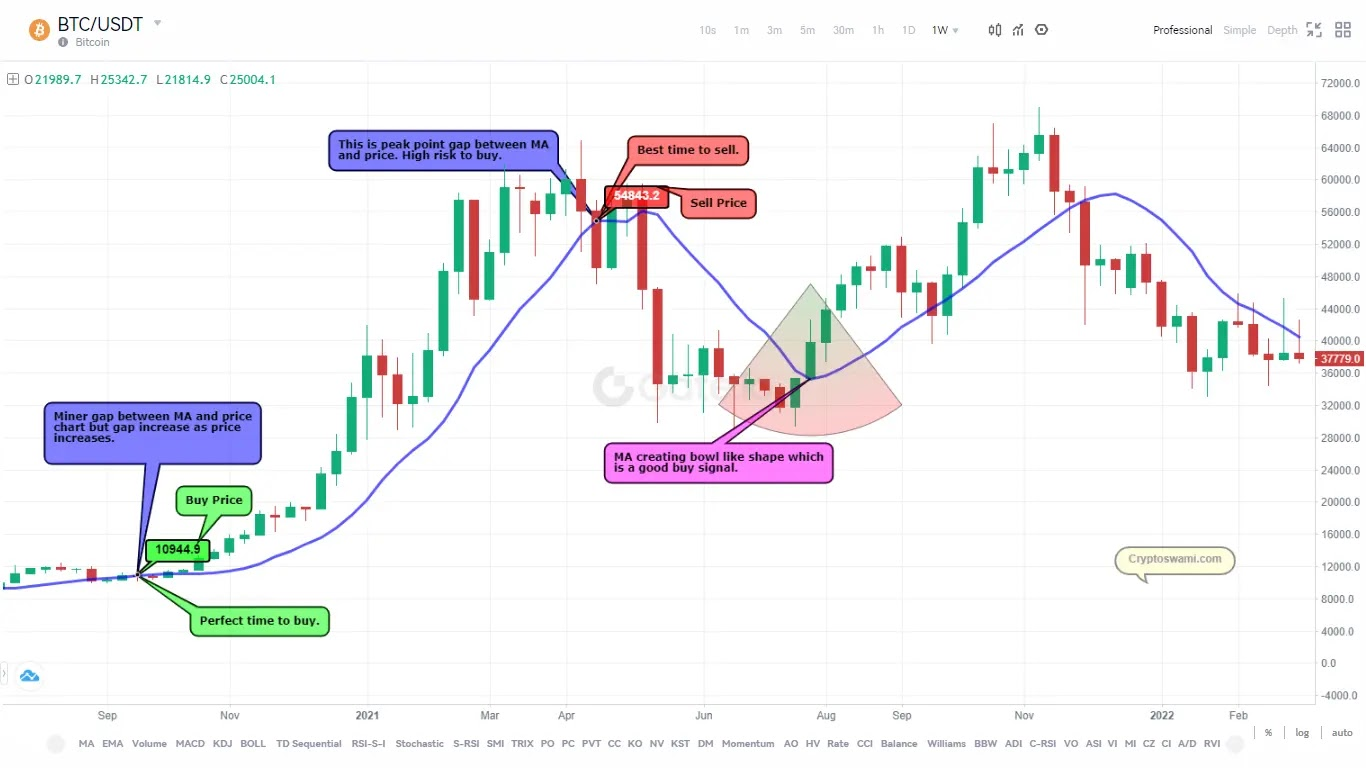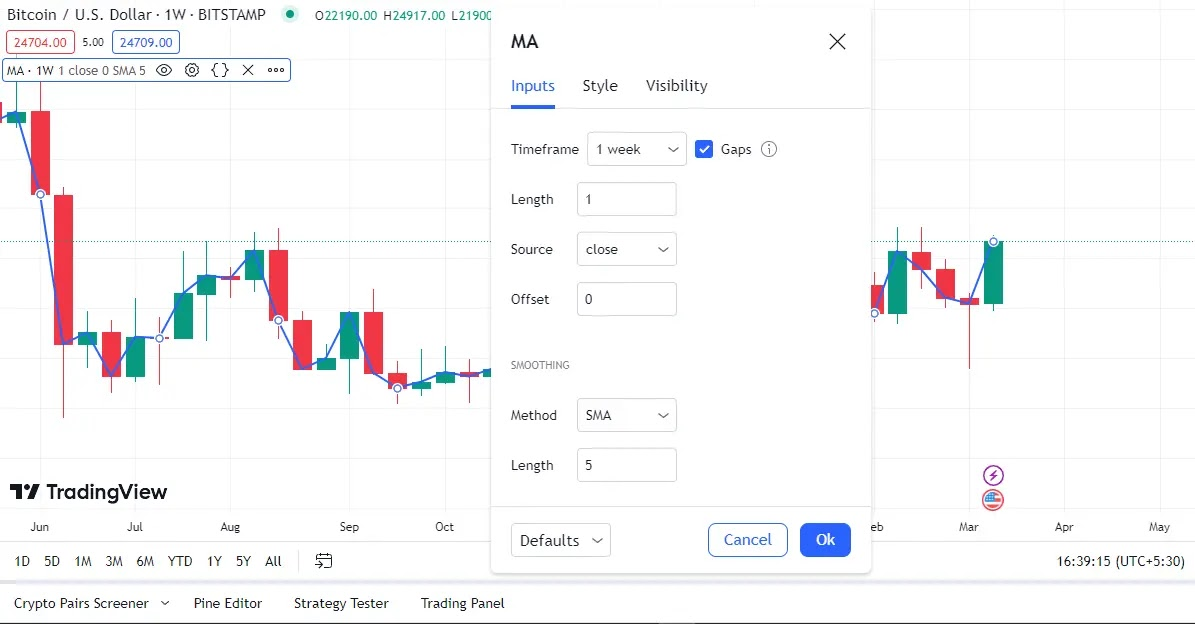# Using a Moving Average To Predict Crypto Market Trends

Moving averages are very common trading tools, whether it is crypto trading or stock trading, and traders take advantage of them very cleverly. But the thing is, in this crowd, can you make some profit by using this moving average indicator in your cryptocurrency trading?

By the way, you will find many articles on the moving average, but they are all based on the stock market. But today we will know what moving averages are and how and when they should be used from a crypto trading perspective.

So let's start.

# What is a moving average?

In simple language, the moving average is an indicator line or point that is used by crypto traders to know the price trend of any crypto. But in technical terms, a moving average is an indicator that shows the change in a crypto's price at a given point in time. The term moving is used because the calculation moves with time. It is also called the moving mean.

A moving average is an indicator that shows the average value of a cryptocurrency's price over a period.

## Types of moving average.

It is mainly of five types: the simple moving average, the exponential moving average, the weighted moving average, the triangular moving average, and the variable moving average. The first and second of these are pretty popular, but which one you can use depends on the type of crypto trading chart you are using.

The choice of moving average type will depend on the specific application and the characteristics of the data being analyzed.Different types of MA. Use one or all.

### Simple Moving Average (MA or SMA).

This is the most popular and basic type of moving average indicator. It consists of a simple line that tells the price of a certain time. It computes the average of a set of data by summing up the values for a specific time period and dividing the sum by the total number of values in that time period.

This type of moving average assigns equal importance to each data point in the time period, regardless of when it occurred.

Formula.
MA = (X1 + X2 + X3 +X4 ……….Xn) / N
Where?
X is the average for the period N.
N is the number of periods.

Example.Let's say there is a trader who wants to trade a Matic Coin, for which he sees the closing price of the coin for the last seven days. And find something like this: \$1, \$1.2, \$1.5, \$0.8, \$2, \$1.7, and \$1. So according to the formula.

MA = (1+1.2+1.5+0.8+2+1.7+1)/7

MA = 1.31 (\$1.31)

### Exponential Moving Average (EMA).

The exponential moving average is quite similar to the weighted moving average, but it gives even more weight to recent data points. This type of moving average uses a formula that assigns exponentially decreasing weights to older data points. It is more responsive to recent changes in the data, making it useful for identifying short-term trends or patterns.

Formula.

It contains a two-step formula. First, find the exponent and then the EMA.

Exp = 2/(Time period + 1)
EMA = (CDP * Exp) + PDA * (1 - Exp)
Where?
Exp = Exponent CDP = Current day price

PDA = Prior Day’s Average (moving average)
Example.

To find the EMA first you have to find the exponent and then calculate \$1, \$1.2, \$1.5, \$0.8, \$2, \$1.7, \$1 (if CDA \$1.5 and PDA \$1.25). So according to the formula.

EXP = 2 / (7+1)
EXP = 0.25
EMA = (1.5 * 0.25) + 1 (1.18 - 0.25)
EMA = 0.375 + 1 * 0.99
EMA = 0.375 + 0.99
EMA = 1.31 (\$1.31)

### Weighted Moving Average (WMA).

The weighted moving average, on the other hand, calculates the average by multiplying each value in the time period by a predetermined weight and adding up the products. Typically, the more recent values are given higher weights, while older values have lower weights. The weighted moving average places more emphasis on more recent data points while assigning less significance to older data points.

## The benefits of using a moving average.

The moving average can protect the trader from price inflation. This can prove to be good for those who are looking for long-term investment opportunities because the more data there is, the better the result will be. Day traders can also use it because the use of a moving average reduces the distraction caused by the often sudden and relatively far-reaching daily price fluctuations, enabling the trader to observe a smoother depiction of the trend changes as they occur.

Identifying Trends.

Moving averages are excellent tools for identifying trends in the crypto market. They help you spot trends by smoothing out price action and allowing you to see the underlying direction of the market. By using moving averages, you can determine whether crypto is trending up, down, or sideways and adjust your trading strategy accordingly.

Entry and Exit Points.

Another benefit of using moving averages is that they can help you determine entry and exit points for your trades. When the price of a cryptocurrency crosses above or below a moving average, it can signal a change in trend, and it may be time to enter or exit a trade. Moving averages can also be used to set stop-loss orders, which help manage risk by limiting potential losses.

Managing Risk.

Managing risk is an essential part of trading stocks. Moving averages can help you manage risk by identifying key support and resistance levels. By placing stop-loss orders at these levels, you can limit your losses if the market moves against you.

## Using the moving average.

This is a simple line, which you can color and structure as per your wish. Although it is used alone with the price chart, if you want, you can also use it with the Bollinger Bands or other indicators. The best time to buy any crypto is when the moving average forms a bowl-like shape around the price line. This is a strong signal that the short-term cycle has bottomed and that prices will move higher.

If the moving average is moving upward, then it is a buy signal, but if the price line moves far away from the moving average, then it is prudent not to buy at that time. On the other hand, if the moving average is coming down, then it would be better not to buy any kind of cryptocurrency. Overall, the best time to buy is when the moving averages are well below or show an identical line for a while.

Remember, whenever the price line gets too far away from the moving averages, it’s time to sell.Using the moving averages to identify trends.

## Why Moving Averages Are Used.

The first purpose of moving averages is to extract data in order to find out what the price trend of a cryptocurrency is. Apart from this, the right time to buy and sell any cryptocurrency can be estimated using the moving average. To get a better data result, you can use a moving average with two indicator lines, one of which will be slower than the other.

## Which type of moving average should you use?

Which type of moving average to use or not depends on which type you specialize in. If experts are to be believed, then the simple moving average is the best, as it is easy to understand and calculate.

## The best time frame for the moving average.

It is difficult to tell because each cryptocurrency has its own trend cycle. But you can choose the time frame according to your trading activity. If you want to do day trading, then 30 days and weekly trading, then 30 weeks' time frame are considered good, but if crypto is of low market cap, then a 200-day moving average time frame is good.

## Setting up the moving average.

In the image below, you can see which data is used to set a moving average.Setting up moving average.

Time frame: First of all, you have to set the time frame, and it is up to you from there until you collect the data. But remember that the time frame of the price chart and the moving average should be the same.

Length: The second is the length by which you calculate the moving average of the back. This is done only when the time frame is large. If the frame is 200 days but I need only 50 days, I will keep the length at 50, and it will automatically calculate the moving average of the last 50 days from the start.

Source: The source also has to be set, like high, low, etc. Basically, it is the price that tells the ups and downs in that time frame. The source is considered good if it is set at an opening or low price.

Offset: An offset refers to the number of data points that are excluded from the calculation of the average. For example, a 10-day moving average with an offset of 3 would only use the data from the 4th day to the 10th day to calculate the average. This means that the first three days of data are excluded from the calculation.

## Conclusion. Moving average.

Do not make mistakes while entering or calculating the moving average data. To get a good result, replace the old data with new data. Like if you are calculating MA for 20 days (from 1st to 20th), then on the 21st day, remove the data of the first day of the last (now you would calculate MA from 2nd to 21st).

And do not copy the data of any cryptocurrency from the internet because the MA of every cryptocurrency changes with time. For safety, you can use more than one moving average, but the data should remain the same. Apart from this, you can also use Bollinger Bands and Stochastic Indicators to strengthen your crypto trading experience. More information you can find here.